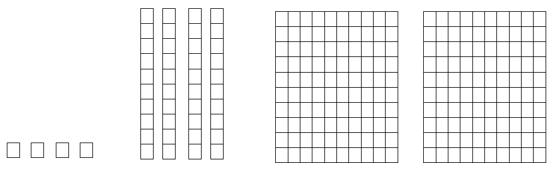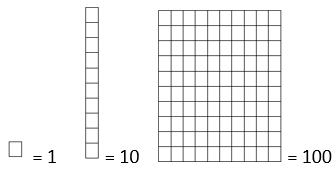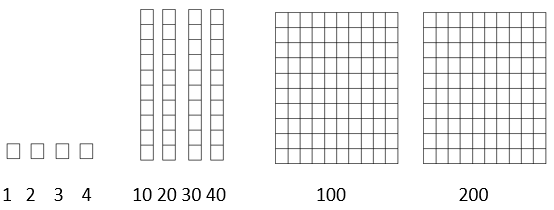# Math Worksheets Land

Math Worksheets For All Ages

# Math Worksheets Land

Math Worksheets For All Ages

# Ones, Tens, Hundreds, and Thousands Blocks Worksheets

What are ones, tens, and hundreds' blocks in math? Learning place values, number concepts, and mathematical operations are the bases of getting a grip on mathematics. A hands-on way of developing a solid understanding of place values is to play around with blocks of ones, tens and hundreds. Blocks can help you develop a thorough understanding of the mathematical concepts, particularly place values. Therefore, it is imperative to understand what are ones, tens, and hundreds' blocks in math. One's blocks - Blocks of ones are single, small blocks of side 1cm each. These blocks look like cubes and provide a hands-on way to solve one-digit sums. They represent the ones/unit position of a digit in a number. Tens blocks - A block of tens is a longer, slender block made up of ten small ones blocks combined or attached together. The dimension of a tens block is; 1 cm x 1 cm x 10 cm. These blocks of tens represent place value of tens in a two or more digits' number. Hundred blocks - A block of hundred is made up of 10 slender blocks of tens or 100 small cubes of ones, making a large flat block of hundreds. This flat, square block measure 1 cm x 10 cm x 10 cm. They are commonly used to represent a hundredth place value in a digit and help in quick additions of numbers. A great selection of worksheets that use place value blocks to help students learn the ones, tens, and hundred place values.

### Aligned Standard: Grade 2 Base Ten - 2.NBT.A.1

• Step-by-step Lesson- The lesson provides a full key and focuses on understanding what each image displays and helps students work through it.
• Guided Lesson - Four problems working up to the hundreds place. You will need to determine how many you have to start and then which digits signify this.
• Guided Lesson Explanation - We first count the number of total values presented and then multiply by their place.
• Practice Worksheet 1 - Follows along with the ones, tens, and hundreds model. This gives you even more practice.
• *Advanced Worksheet - This moves to the thousands place. This is outside of the scope of the standard.
• Answer Keys - These are for all the unlocked materials above.

### Working with Ones, Tens, and Hundreds Blocks Sheets

• Practice 2 - Find the value of each group of base 10 blocks.
• Practice 3 - Another version with many more hundreds places.
• Practice 4 - Okay, there are just too many hundreds places here.

### *Ones, Tens, Hundreds, and Thousands Blocks Practice Worksheets* - Beyond Standard

• Practice 2- Use the legend to get you started here.
• Practice 3- We introduce the thousands place here.
• Practice 4- This one walks you in nicely. Take your time and get going with it.

### Skill Quizzes

• Blocks Quiz 1- The totals here are very large. Which make it a good assessment of where you are.
• Practice Quiz 2- Base ten all comes from this skill. We ask you to bring this all to the wall for us.
• Practice Quiz 3- This is the easiest of the bunch. Nice to see where you are at with it though.

### Example Problem

Write the value that the number blocks found below indicate.We have 3 sets of number blocks. The individual blocks indicate digits found at the ones place. The single column, ten row blocks indicate the values of the tens place. the ten column, ten row block indicates the value of the hundred place. The chart below describes each type of block:To solve this problem and best understand the values that are present it is helpful to number each of the blocks in the successive number that they represent. We have labelled what each and every block represents below.We can simply convert these values to the expand form of a number. In this case we have 2 hundreds, 4 tens, and 4 ones or 200 + 40 + 4. All we need to do now is find the end sum. 200 + 40 + 4 = 244

## Why Is Understanding These Block Important?

The base ten mathematical system we use very intuitive and helpful in all walks of life. The basic concept of what one place to the next indicates is amazingly easy to understand when it is seen visually. Researchers from several students have found that using manipulatives and visual models when teaching this topic help to make a substantial difference for prolonged learning. The end goal is for students to understand that they is a large difference between values (ten times as much) from place to place. This also helps students understand that every zero you put on the end of your paycheck is ten times greater.

Unlock all the answers, worksheets, homework, tests and more!
Save Tons of Time! Make My Life Easier Now

## Thanks and Don't Forget To Tell Your Friends!

I would appreciate everyone letting me know if you find any errors. I'm getting a little older these days and my eyes are going. Please contact me, to let me know. I'll fix it ASAP.